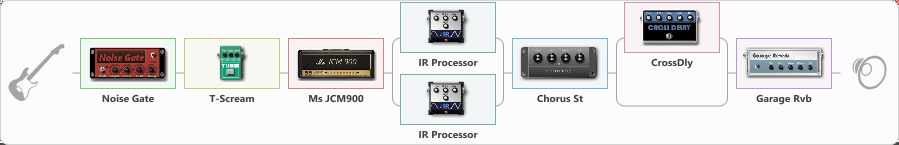# Tommy heavy par

Discussion in 'ToneLib-GFX presets' started by truss van halen, Dec 6, 2020.

1. ### truss van halenWell-Known Member

Tommy heavy par

Preset name: Tommy heavy par

Effects chain:Effect: "Noise Gate" (Dynamics / Filter), active - "yes"
{
"Mode" = Manual
"Depth" = 50
"Threshold" = 50
"Attack" = 0
"Hold" = 5
"Decay" = 250
}

Effect: "T-Scream" (Overdrive / Distortion), active - "yes"
{
"Drive" = 36
"Tone" = 80
"Level" = 79
}

Effect: "Ms JCM900" (Amp simulators), active - "yes"
{
"Gain" = 80
"Bass" = 56
"Middle" = 69
"Treble" = 65
"Presence" = 63
"Master" = 47
"Level (dB)" = 7
}

Effect: "Splitter" (Dynamics / Filter), active - "yes"
{
"A-Bypass" = Off
"A-Pan" = 0
"A-Level" = 55
"B-Bypass" = Off
"B-Pan" = 0
"B-Level" = 55

'A' branch:
{

Effect: "IR Processor" (Cabinets), active - "yes"
{
"IR" = Cab IR Sample3
"Low Cut (Hz)" = 0
"Hi Cut (kHz)" = 20.0
"Mix" = 100
"Level (dB)" = 0
}
}
'B' branch:
{

Effect: "IR Processor" (Cabinets), active - "yes"
{
"IR" = Cab IR Sample2
"Low Cut (Hz)" = 0
"Hi Cut (kHz)" = 6.0
"Mix" = 100
"Level (dB)" = 0
}
}
}

Effect: "Chorus St" (Modulation / Sfx), active - "yes"
{
"Speed" = 1.9
"Depth" = 31
"Center" = 4.4
"Mix" = 40
}

Effect: "Splitter" (Dynamics / Filter), active - "yes"
{
"A-Bypass" = Off
"A-Pan" = 0
"A-Level" = 55
"B-Bypass" = Off
"B-Pan" = 0
"B-Level" = 55

'A' branch:
{

Effect: "CrossDly" (Delay), active - "yes"
{
"Time" = Note sync, 1/4
"Feedback" = 44
"Tone" = 76
"Sens" = 0
"Mix" = 58
}
}
'B' branch:
{
}
}

Effect: "Garage Rvb" (Reverberation), active - "yes"
{
"Time" = 5.2
"PreDelay" = 46
"LoDamp" = 0
"HiDamp" = 30
"Mix" = 10
}

Note: You will need to download and install the ToneLib-GFX software to use the preset.

File size:
5.2 KB
Views:
2,520